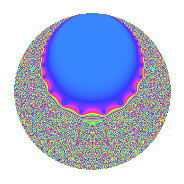# Properties

 Label 4.19.bLevel 4 Weight 19 Character orbit b Rep. character $$\chi_{4}(3,\cdot)$$ Character field $$\Q$$ Dimension 8 Newforms 1 Sturm bound 9 Trace bound 0

# Related objects

## Defining parameters

 Level: $$N$$ = $$4 = 2^{2}$$ Weight: $$k$$ = $$19$$ Character orbit: $$[\chi]$$ = 4.b (of order $$2$$ and degree $$1$$) Character conductor: $$\operatorname{cond}(\chi)$$ = $$4$$ Character field: $$\Q$$ Newforms: $$1$$ Sturm bound: $$9$$ Trace bound: $$0$$

## Dimensions

The following table gives the dimensions of various subspaces of $$M_{19}(4, [\chi])$$.

Total New Old
Modular forms 10 10 0
Cusp forms 8 8 0
Eisenstein series 2 2 0

## Trace form

 $$8q$$ $$\mathstrut +\mathstrut 84q^{2}$$ $$\mathstrut +\mathstrut 352016q^{4}$$ $$\mathstrut +\mathstrut 860880q^{5}$$ $$\mathstrut +\mathstrut 310944q^{6}$$ $$\mathstrut -\mathstrut 154160064q^{8}$$ $$\mathstrut -\mathstrut 729541560q^{9}$$ $$\mathstrut +\mathstrut O(q^{10})$$ $$8q$$ $$\mathstrut +\mathstrut 84q^{2}$$ $$\mathstrut +\mathstrut 352016q^{4}$$ $$\mathstrut +\mathstrut 860880q^{5}$$ $$\mathstrut +\mathstrut 310944q^{6}$$ $$\mathstrut -\mathstrut 154160064q^{8}$$ $$\mathstrut -\mathstrut 729541560q^{9}$$ $$\mathstrut -\mathstrut 853591160q^{10}$$ $$\mathstrut -\mathstrut 2983758720q^{12}$$ $$\mathstrut -\mathstrut 6658778288q^{13}$$ $$\mathstrut -\mathstrut 3330240576q^{14}$$ $$\mathstrut +\mathstrut 35282993408q^{16}$$ $$\mathstrut +\mathstrut 213854181648q^{17}$$ $$\mathstrut +\mathstrut 197420552436q^{18}$$ $$\mathstrut -\mathstrut 421824543840q^{20}$$ $$\mathstrut -\mathstrut 771822339072q^{21}$$ $$\mathstrut -\mathstrut 586750684320q^{22}$$ $$\mathstrut -\mathstrut 3940602195456q^{24}$$ $$\mathstrut +\mathstrut 528925732440q^{25}$$ $$\mathstrut +\mathstrut 12314194404552q^{26}$$ $$\mathstrut -\mathstrut 20860043224320q^{28}$$ $$\mathstrut +\mathstrut 11238056568912q^{29}$$ $$\mathstrut +\mathstrut 63814514963520q^{30}$$ $$\mathstrut -\mathstrut 133632603995136q^{32}$$ $$\mathstrut -\mathstrut 21541938424320q^{33}$$ $$\mathstrut +\mathstrut 295093712425768q^{34}$$ $$\mathstrut -\mathstrut 595880470532208q^{36}$$ $$\mathstrut -\mathstrut 158886968816432q^{37}$$ $$\mathstrut +\mathstrut 830666716492320q^{38}$$ $$\mathstrut -\mathstrut 1609388875268480q^{40}$$ $$\mathstrut +\mathstrut 451509984725136q^{41}$$ $$\mathstrut +\mathstrut 3247297173265920q^{42}$$ $$\mathstrut -\mathstrut 3429204744604800q^{44}$$ $$\mathstrut +\mathstrut 394282398204240q^{45}$$ $$\mathstrut +\mathstrut 3658225092496704q^{46}$$ $$\mathstrut -\mathstrut 6713415182530560q^{48}$$ $$\mathstrut -\mathstrut 2251201133570680q^{49}$$ $$\mathstrut +\mathstrut 6942506471898780q^{50}$$ $$\mathstrut -\mathstrut 6479715597253472q^{52}$$ $$\mathstrut +\mathstrut 1216384760086992q^{53}$$ $$\mathstrut +\mathstrut 3284600487812928q^{54}$$ $$\mathstrut +\mathstrut 7432202560564224q^{56}$$ $$\mathstrut +\mathstrut 2661563378188800q^{57}$$ $$\mathstrut -\mathstrut 17440122911455928q^{58}$$ $$\mathstrut +\mathstrut 36392091128935680q^{60}$$ $$\mathstrut +\mathstrut 184592915104336q^{61}$$ $$\mathstrut -\mathstrut 42580340982416640q^{62}$$ $$\mathstrut +\mathstrut 74097564160495616q^{64}$$ $$\mathstrut -\mathstrut 5670291051587040q^{65}$$ $$\mathstrut -\mathstrut 140809597617788160q^{66}$$ $$\mathstrut +\mathstrut 131212356714741792q^{68}$$ $$\mathstrut -\mathstrut 26839260584143872q^{69}$$ $$\mathstrut -\mathstrut 153862868594448000q^{70}$$ $$\mathstrut +\mathstrut 156072744876684864q^{72}$$ $$\mathstrut +\mathstrut 31494366486758032q^{73}$$ $$\mathstrut -\mathstrut 108949653815722872q^{74}$$ $$\mathstrut +\mathstrut 152098090223483520q^{76}$$ $$\mathstrut +\mathstrut 180811187963888640q^{77}$$ $$\mathstrut +\mathstrut 14085206442507840q^{78}$$ $$\mathstrut -\mathstrut 152292076037383680q^{80}$$ $$\mathstrut -\mathstrut 260466732987857784q^{81}$$ $$\mathstrut +\mathstrut 335145451981844968q^{82}$$ $$\mathstrut -\mathstrut 850166994683553792q^{84}$$ $$\mathstrut -\mathstrut 204660361893474400q^{85}$$ $$\mathstrut +\mathstrut 950236163482864224q^{86}$$ $$\mathstrut -\mathstrut 1369014843355476480q^{88}$$ $$\mathstrut +\mathstrut 162001265029847952q^{89}$$ $$\mathstrut +\mathstrut 1771450132254688200q^{90}$$ $$\mathstrut -\mathstrut 1193579560451823360q^{92}$$ $$\mathstrut +\mathstrut 668158876340367360q^{93}$$ $$\mathstrut +\mathstrut 1478730179820369024q^{94}$$ $$\mathstrut -\mathstrut 2028358051400441856q^{96}$$ $$\mathstrut -\mathstrut 1214976752903766512q^{97}$$ $$\mathstrut +\mathstrut 2427758859173433876q^{98}$$ $$\mathstrut +\mathstrut O(q^{100})$$

## Decomposition of $$S_{19}^{\mathrm{new}}(4, [\chi])$$ into irreducible Hecke orbits

Label Dim. $$A$$ Field CM Traces $q$-expansion
$$a_2$$ $$a_3$$ $$a_5$$ $$a_7$$
4.19.b.a $$8$$ $$8.215$$ $$\mathbb{Q}[x]/(x^{8} - \cdots)$$ None $$84$$ $$0$$ $$860880$$ $$0$$ $$q+(11-\beta _{1})q^{2}-\beta _{2}q^{3}+(44007-9\beta _{1}+\cdots)q^{4}+\cdots$$